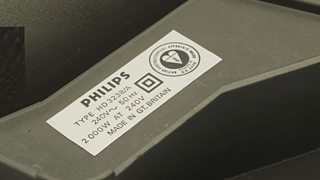# Electrical power

Electrical energy itself is not a useful form of energy for us, but it supplies energy to our appliances which transform it into other forms of energy which are useful.

An electric fire, a cooker, a toaster and an electric kettle all convert electrical energy into heat energy.

The of an appliance is the rate at which energy is transferred.

• $Power = \frac{{energy}}{{time}}$
• $P = \frac{E}{t}$
• The symbol for power is $$P$$, it is measured in $$(W)$$
• The symbol for energy is $$E$$, it is measured in joules $$(J)$$
• The symbol for time is $$t$$, it is measured in seconds $$(s)$$

Appliances in the home have their power ratings stated on their base plates:Power rating plate on electrical apparatus

Typical power ratings include:

AppliancePower rating $(W)$
Kettle2000
Microwave oven (cooking power of $800$)1000
Toaster900
Hair dryer1200
Fridge135

## Example

Question

Calculate the power rating of a hair dryer that is used for 2 minutes and uses 100 kilojoules of energy.

To calculate the power rating you need to take the following steps:

$power = energy \div time$

$= 100000 \div (2 \times 60)$

$= 830 watts\,(830 W)$

$Energy = 830 W$